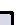Войти в системуHome    - Создать дневник    - Написать в дневник       - Подробный режимLJ.Rossia.org    - Новости сайта    - Общие настройки    - Sitemap    - Оплата    - ljr-fifРедактировать...    - Настройки    - Список друзей    - Дневник    - Картинки    - Пароль    - Вид дневникаСообществаНастроить S2Помощь    - Забыли пароль?    - FAQ    - Тех. поддержкаПишет Misha Verbitsky ()@ 2019-11-01 00:10:00Настроение:sick Музыка: A geometric flow of Balanced metrics Entry tags: math

a (non isotrivial) tori fibration
Запишу сей пример, чтобы не потерялся.

>Let Y be a compact complex simply connected projective manifold and W a
>non trivial holomorphic vector bundle of rank n. Assume that there are
>2n R-linearly independent holomorphic sections of W and take the
>quotient by the corresponding action of Z^{2n}. This gives a fibration
>f : X \to Y with fibers non isotrivial complex tori.

>...is it possible to have such a (non isotrivial) tori
>fibration with the total space X having trivial canonical class K_X
>(or equivalently K_Y being trivial)?

This is an interesting construction, say, it works for the
twistor space of a torus. Not sure there are other examples,
not related to the twistor spaces.

Here is a Calabi-Yau example: take a hyperelliptic curve C of
genus 4, and let $\mu:\; C \arrow \C P^1$ be a 2-sheeted covering.
Let $\pi:\; Tw\arrow \C P^1$ be the twistor space of a
complex torus $T^4$ of real dimension 4. Then the fibered product
$F:=C \times_{\C P^1} Tw$ is $T^4$-fibration over C which satisfies
your assumptions: it is a non-isotrivial smooth toric fibration,
and its canonical bundle is a product of $K_C$ and $\mu^*(O(1))$.
The canonical bundle of $F$ is a pullback of a certain line bundle
L on C, with $L= K_C \otimes \mu^*(O(2))$. As explained here
https://math.stackexchange.com/questions/1592977/divisors-of-degree-2g-2-on-a-hyperelliptic-curve-of-genus-g
$L$ is acctually trivial (they explain that any
degree $2g-2$ divisor on an hyperelliptic curve which is invariant
under the standard involution is canonical divisor)

* * *

Вроде нигде не наврал.

Привет